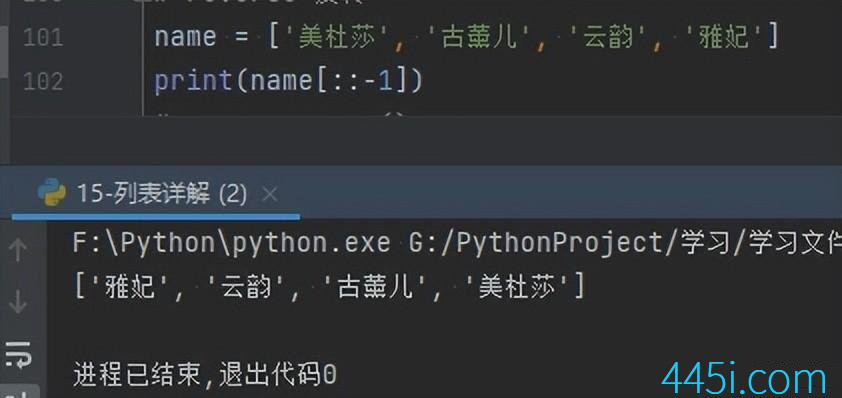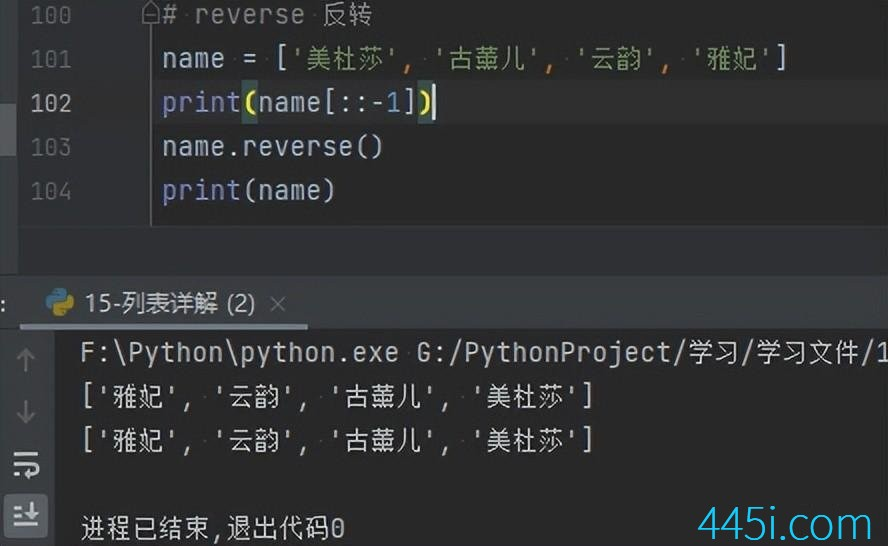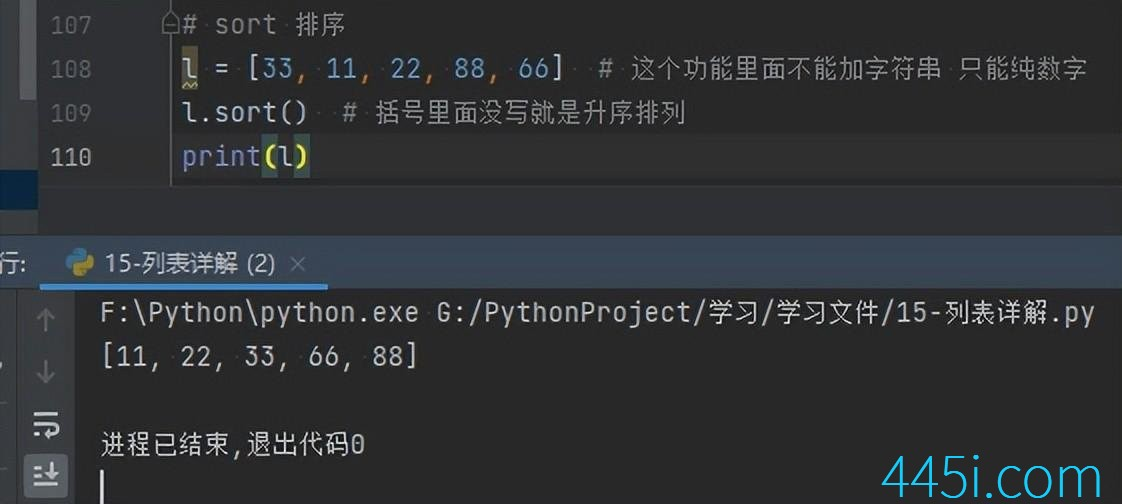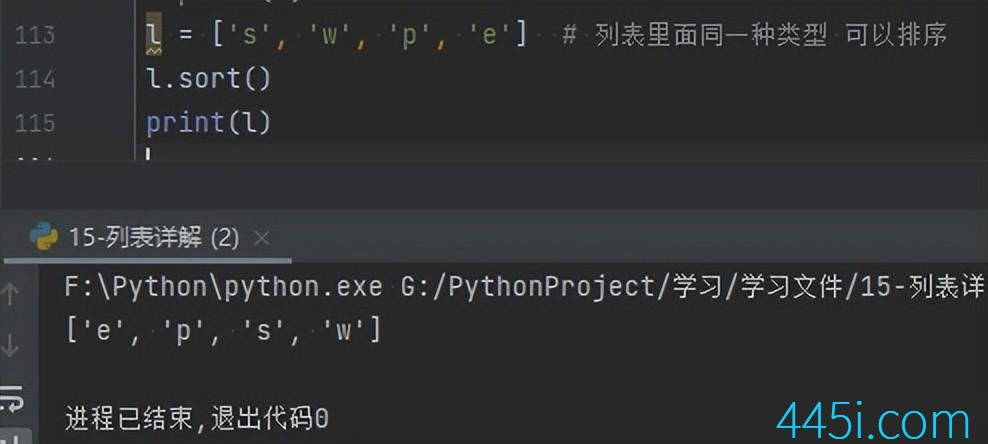# Python 列表详解

``````name = ['美杜莎', '古薰儿', '云韵', '雅妃','美杜莎','美杜莎','美杜莎']
name.clear()
print(name)````````````name = ['美杜莎', '古薰儿', '云韵', '雅妃']
print(name[::-1])````````````name = ['美杜莎', '古薰儿', '云韵', '雅妃']
print(name[::-1])
name.reverse()
print(name)````````````l=[33,11,22,88,66]
l.sort()
print(l)``````reverse本身也是反转的意思，就相当于在原来的基础上反转一下。我们来运行看看。

``````l = [33, 11, 22, 88, 66]
l.sort(reverse=True)
print(l)````````````l=['s','w','p','e'] # 列表里面同一种类型 可以排序
l.sort()
print(l)``````### 觉得文章有用就打赏一下文章作者

#### 支付宝扫一扫打赏#### 微信扫一扫打赏